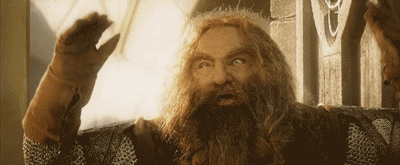# interactive and styled middle earth map

• December 2016
• R

This is pure nerdism. There is a project to create a shapfile from a fictional world, the Middle Earth. The data is in this https://github.com/jvangeld/ME-GIS repository. The author of the r-chart.com web site made a ggplot version of this map which you can see in this link.

Well, as the highcharter developer I wanted to try to made this map using this package and add some styles to give the old magic fashioned Middle Earth look. My try to achieve this…Just because we can – Me.

… Is summarized as:

• Use the `#F4C283` color as background.
• use the Tangerine font in the title and Macondo in the legends, tooltips and labels.
• Search hexadecimal color forest to get the the colors for forests.

The packages to made this possible were:

``````rm(list = ls())
library(dplyr)
library(maptools)
library(highcharter)
library(geojsonio)
library(rmapshaper)
``````

Note we used the `rmapshaper` package (wapper for the mapshaper js library) to simplify the rivers beacuse this file is kind of huge to put in a htmlwidget.

I made some auxiliar functions to simplify the shapefile and to converte this info in geojson format using the geojsonio package.

``````fldr <- "~/ME-GIS-master"

shp_to_geoj_smpl <- function(file = "Coastline2.shp", k = 0.5) {
d <- readShapeSpatial(file.path(fldr, file))
d <- ms_simplify(d, keep = k)
d <- geojson_list(d)
d
}

shp_points_to_geoj <- function(file) {
outp <- readShapeSpatial(file.path(fldr, file))
outp <- geojson_json(outp)
outp
}

cstln <- shp_to_geoj_smpl("Coastline2.shp", .65)
rvers <- shp_to_geoj_smpl("Rivers19.shp", .01)
frsts <- shp_to_geoj_smpl("Forests.shp", 0.90)
lakes <- shp_to_geoj_smpl("Lakes2.shp", 0.1)

cties <- shp_points_to_geoj("Cities.shp")
towns <- shp_points_to_geoj("Towns.shp")

pointsyles <- list(
symbol = "circle",
lineWidth= 1,
fillColor= "transparent",
lineColor= NULL
)
``````

Now, to create the chart we need to add the geographic info one by one setting the type of info:

• `mappoint` for cities.
• `mapline` for rivers and the coast.
• And `map` for lakes and forests.
``````hcme <- highchart(type = "map") %>%
hc_chart(style = list(fontFamily = "Macondo"), backgroundColor = "#F4C283") %>%
hc_title(text = "The Middle Earth", style = list(fontFamily = "Tangerine", fontSize = "40px")) %>%
hc_add_series(data = cstln, type = "mapline", color = "brown", name = "Coast") %>%
hc_add_series(data = rvers, type = "mapline", color = "#7e88ee", name = "Rivers") %>%
hc_add_series(data = roads, type = "mapline", color = "#634d53", name = "Main Roads") %>%
hc_add_series(data = frsts, type = "map", color = "#228B22", name = "Forest") %>%
hc_add_series(data = lakes, type = "map", color = "#7e88ee", name = "Lakes") %>%
hc_add_series(data = cties, type = "mappoint", color = "black", name = "Cities",
dataLabels = list(enabled = TRUE), marker = list(radius = 4, lineColor = "black")) %>%
hc_add_series(data = towns, type = "mappoint", color = "black", name = "Towns",
dataLabels = list(enabled = TRUE), marker = list(radius = 1, fillColor = "rgba(190,190,190,0.7)")) %>%
hc_plotOptions(
series = list(
marker = pointsyles,
tooltip = list(headerFormat = "", poinFormat = "{series.name}"),
dataLabels = list(enabled = FALSE, format = '{point.properties.Name}')
)
) %>%
``````

And you have a super styled chart using only R :D!

``````hcme
``````

open

If you deselect the `Towns` series the chart will zoom smoothly. There are some many point that you can see the chart/browser lag when you make a zoom.source